Dr. J's Maths.com
Where the techniques of Maths
are explained in simple terms.

Trigonometry - auxilliary angles.
Test Yourself 1.

 Questions on this page address: 1. Simplification of basic expressions. 2. Solving equations requiring the conversion to auxilliary angle format. 3. Solving problems incorporating the auxilliary angle format.

 Simplify the following basic expressions. 1. Write the expression sin x - cos x in the form A sin (x - β). Answer.√2sin (x - π/4). 2. Write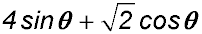in the form Rsin (θ + α). (α in degrees). Answer.3√2sin (x + 19° 28'). 3. (i) Write f(A) = 4cos A - 3 sin A in the form R cos (A + θ) - express θ to the nearest degree. (ii) Hence sketch f(A) for 0° < A < 360°. Answer.R = 5 and θ = 37°. 4. Given 2cos(x + β) = cosx - Asinx where 0 ≤ β ≤ π/2, find the values of A and β. Answer.A = √3 and β = 60°. 5. Sketch the curve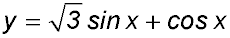by first expressing it in the form A sin(x + α). Answer.A = 2 and α = π/6. 6. (i) Express sin x + cos x in the form A sin (x + β). (ii) Hence sketch y = sin x + cos x(for -π ≤ x ≤ 2π)? (iii) How many solutions are there for sin x + cos x = 1 within that domain? Answer.(i) A = √2 and α = π/6. (iii) There are 3 solutions in that domain. 7. What is the maximum value of 5 sin 4t + 12cos 4t? Answer.Max = 13. 8. (i) Find the coordinates of the points where the maximum and minumum values of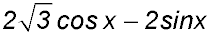occur. (ii) Sketch the curve for 0° ≤ x ≤ 2π . Answer.Max at (11π/6, 4); min at (5π/6, -4). Solve equations. 9. (i) Write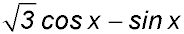in the form 2cos(x + β) where 0 ≤ β ≤ π/2. (ii) Hence or otherwise solve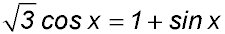for 0 ≤ β ≤ 2π. Answer.(i) 2cos(x + π/6)(ii) x = π/6 or x = 3π/2. 10. (i) Express 3 cosx - 4 sinx in the form A cos (x + α) for some R > 0 and 0 ≤ α ≤ 90° giving α correct to the nearest minute. (ii) Hence solve the equation 3 cos γ - 4 sin γ - 5 = 0 giving your answer to the nearest minute (0° < γ < 360°). Answer.γ = 53° 8'. 11. Solve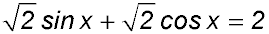(for -2π ≤ x≤ 2π). Answer.x= -7π/4 or π/4. 12. Solve 6 sinx + 8 cosx + 5 = 0 for 0° < x < 360°. Answer.x = 156°52' or 276° 52'. 13. Solve for 0° ≤ ψ ≤ 360° (to the nearest minute): 2cos ψ + 4sinψ = 4. Answer.ψ = 90°. 14. Solve the equation 8sin 2θ + 15 cos 2θ = 17 by first writing it in the form A sin (2θ + β) for 0° < θ < 360°. Answer.θ = 14° 2' or 194° 2'. 15. Find all angles θ where 0 ≤ θ ≤ 2π for which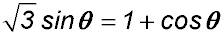. Answer.π/3 and π. Solve problems. 16. A position of a particle moving along a straight line can be described by the equation P = 3 sint + 4cost where t is the time (in seconds) since the particle began to move. (i) Express the equation for P in the form Rcos(t - α) where R > 0 and 0 < α < π/2 giving the value of α in radians to 3 decimal places. (ii) Hence find the values of t, correct to the nearest tenth of a second, to indicate when the particle first reaches its maximum and minimum distances from the origin. Also state those distances. Answer.(i) Max of 5 at 0.644 secs.(ii) Min of -5 at 3.785 secs. 17. The height of a wave at the beach where a surfing competition is to be held is determined by one of the surfers to be estimated by the equation h = 5cos 0.5t + 12 sin 0.5t where t is time in hours. (i) Another surfer claims it is better to redefine the height equation in the form Asin (0.5t + β). Find the values for A and β which will allow that alternative format for the height equation. (ii) What is the initial height of the tide? (iii) Find the first two values for t (t > 0 hours) when the wave reaches a height of 10 metres. Answer.(ii) Initial height is 12 m. (iii) 10 metres at 2.18 hours and 11.97 hours. 18.(i) Express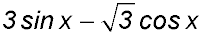in the form Asin (x - α) where A > 0 and 0 ≤ α ≤ π/2. (ii) Determine the minimum value for. (iii) Solve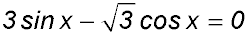for 0 ≤ x ≤ 2π. Answer.(ii) Minimum at -2√3. (iii) x = 5π/6 and 11π/6. 19. A particle moves in a straight line with its position at any time t mins described by x = 3cos 2t + 4sin 2t. (i) Develop an equation in the form A cos (2t + β) to describe its velocity. (ii) Hence find the first two occasions when the particle is at rest. Answer.(i) Vel = 10 cos(2t + 0.643). (ii) At rest at 27.5 secs andat 2 mins 2 secs. 20. The motion of a particle can be expressed as Asin (t + B). Separating this composite equation into its components results in the form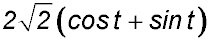. Find the values of A and B. Answer.A=10 and B = π/4.